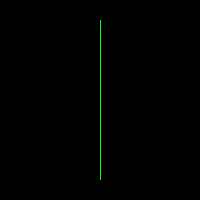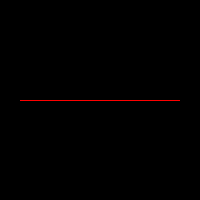# Create and image and draw a line in Rust

In this example we continue the cration of simple images using the image Rust crate.

We started with an empty image and now with this code we created two small images and drew two lines:examples/create-image-draw-line/src/main.rs

``````use image::{RgbImage, ImageBuffer};

fn main() {
red_horizontal_line();
green_vertical_line();
}

fn red_horizontal_line() {
let width = 200;
let height = 200;

let red = 255 as u8;
let green = 0;
let blue = 0;

let mut img: RgbImage = ImageBuffer::new(width, height);
let y = 100;
for x in 20..180 {
*img.get_pixel_mut(x, y) = image::Rgb([red, green, blue]);
}

img.save("red_horizontal_line.png").unwrap();
}

fn green_vertical_line() {
let width = 200;
let height = 200;

let red = 0 as u8;
let green = 255;
let blue = 0;

let mut img: RgbImage = ImageBuffer::new(width, height);
let x = 100;
for y in 20..180 {
*img.get_pixel_mut(x, y) = image::Rgb([red, green, blue]);
}

img.save("green_vertical_line.png").unwrap();
}

``````